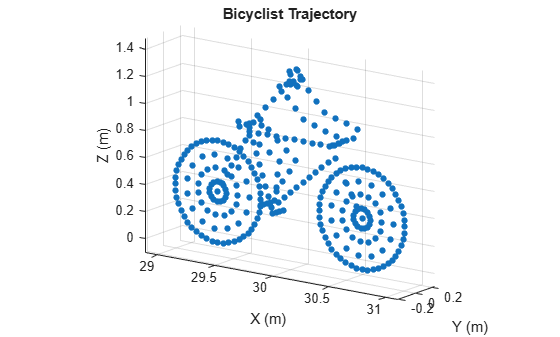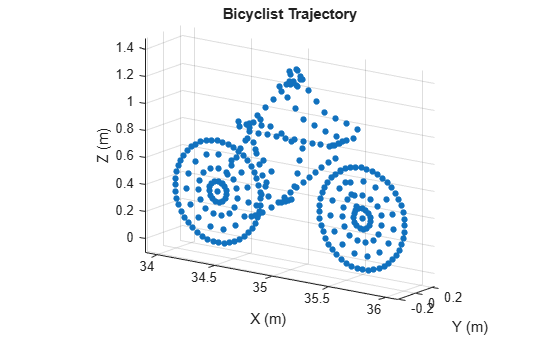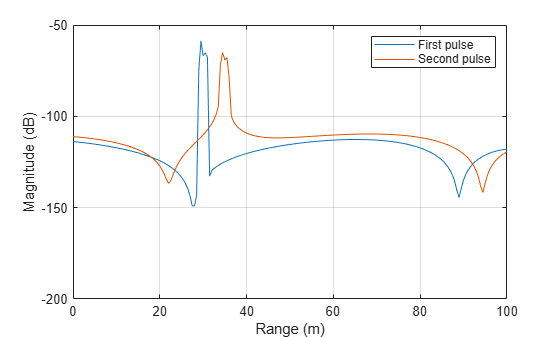# reflect

Reflected signal from moving bicyclist

## Syntax

``Y = reflect(bicyclist,X,ang)``

## Description

example

````Y = reflect(bicyclist,X,ang)` returns the total reflected signal, `Y`, from a bicyclist. The total reflected signal is the sum of all reflected signals from the bicyclist scatterers. `X` represents the incident signals at each scatterer. `ang` defines the directions of the incident and reflected signals with respect to the each scatterers.The reflected signal strength depends on the value of the radar cross-section at the incident angle. This simplified model uses the same value for all scatterers.```

## Examples

collapse all

Compute the backscattered radar signal from a bicyclist moving along the x-axis at 5 m/s away from a radar. Assume that the radar is located at the origin. The radar transmits an LFM signal at 24 GHz with a 300 MHz bandwidth. A signal is reflected at the moment the bicyclist starts to move and then one second later.

Initialize Bicyclist, Waveform, and Propagation Channel Objects

Initialize the `backscatterBicyclist`, `phased.LinearFMWaveform`, and `phased.FreeSpace` objects. Assume a 300 MHz sampling frequency. The initial position of the bicyclist lies on the x-axis 30 meters from the radar.

```bw = 300e6; fs = bw; fc = 24e9; radarpos = [0;0;0]; bpos = [30;0;0]; bicyclist = backscatterBicyclist( ... 'OperatingFrequency',fc,'NumWheelSpokes',15, ... 'InitialPosition',bpos,'Speed',5.0, ... 'InitialHeading',0.0); lfmwav = phased.LinearFMWaveform( ... 'SampleRate',fs, ... 'SweepBandwidth',bw); sig = lfmwav(); chan = phased.FreeSpace( ... 'OperatingFrequency',fc, ... 'SampleRate',fs, ... 'TwoWayPropagation',true);```

Plot Initial Bicyclist Position

Using the `move` object function, obtain the initial scatterer positions, velocities and the orientation of the bicyclist. Plot the initial position of the bicyclist. The `dt` argument of the `move` object function determines that the next call to `move` returns the bicyclist state of motion `dt` seconds later.

```dt = 1.0; [bpos,bvel,bax] = move(bicyclist,dt,0); plot(bicyclist)```Obtain First Reflected Signal

Propagate the signal to all scatterers and obtain the cumulative reflected return signal.

```N = getNumScatterers(bicyclist); sigtrns = chan(repmat(sig,1,N),radarpos,bpos,[0;0;0],bvel); [rngs,ang] = rangeangle(radarpos,bpos,bax); y0 = reflect(bicyclist,sigtrns,ang);```

Plot Bicyclist Position After Position Update

After the bicyclist has moved, obtain the scatterer positions and velocities and then move the bicycle along its trajectory for another second.

```[bpos,bvel,bax] = move(bicyclist,dt,0); plot(bicyclist)```Obtain Second Reflected Signal

Propagate the signal to all scatterers at their new positions and obtain the cumulative reflected return signal.

```sigtrns = chan(repmat(sig,1,N),radarpos,bpos,[0;0;0],bvel); [~,ang] = rangeangle(radarpos,bpos,bax); y1 = reflect(bicyclist,sigtrns,ang);```

Match Filter Reflected Signals

Match filter the reflected signals and plot them together.

```mfsig = getMatchedFilter(lfmwav); nsamp = length(mfsig); mf = phased.MatchedFilter('Coefficients',mfsig); ymf = mf([y0 y1]); fdelay = (nsamp-1)/fs; t = (0:size(ymf,1)-1)/fs - fdelay; c = physconst('LightSpeed'); plot(c*t/2,mag2db(abs(ymf))) ylim([-200 -50]) xlabel('Range (m)') ylabel('Magnitude (dB)') ax = axis; axis([0,100,ax(3),ax(4)]) grid legend('First pulse','Second pulse')```Compute the difference in range between the maxima of the two pulses.

```[maxy,idx] = max(abs(ymf)); dpeaks = t(1,idx(2)) - t(1,idx(1)); drng = c*dpeaks/2```
```drng = 4.9965 ```

The range difference is 5 m, as expected given the bicyclist speed.

## Input Arguments

collapse all

Bicyclist, specified as a `backscatterBicyclist` object.

Incident radar signals on each bicyclist scatterer, specified as a complex-valued M-by-N matrix. M is the number of samples in the signal. N is the number of point scatterers on the bicyclist and is determined partly from the number of spokes in each wheel, Nws. See Bicycle Scatterer Indices for the column representing the incident signal at each scatterer.

The size of the first dimension of the input matrix can vary to simulate a changing signal length. A size change can occur, for example, in the case of a pulse waveform with variable pulse repetition frequency.

Data Types: `double`
Complex Number Support: Yes

Directions of incident signals on the bicyclist scatterers, specified as a real-valued 2-by-N matrix. N equals the number of columns in `X`. Each column of `Ang` specifies the incident direction of the signal to a scatterer taking the form of an azimuth-elevation pair, [AzimuthAngle;ElevationAngle]. Units are in degrees. See Bicycle Scatterer Indices for the column representing the incident direction at each scatterer.

Data Types: `double`

## Output Arguments

collapse all

Total reflected radar signals, returned as a complex-valued M-by-1 column vector. M equals the number of samples in the input signal, `X`.

Data Types: `double`
Complex Number Support: Yes

collapse all

### Bicycle Scatterer Indices

Bicyclist scatterer indices define which columns in the scatterer position or velocity matrices contain the position and velocity data for a specific scatterer. For example, column 92 of `bpos` specifies the 3-D position of one of the scatterers on a pedal.

The wheel scatterers are equally divided between the wheels. You can determine the total number of wheel scatterers, N, by subtracting 113 from the output of the `getNumScatterers` function. The number of scatterers per wheel is Nsw = N/2.

Bicyclist Scatterer Indices

Bicyclist ComponentBicyclist Scatterer Index
Frame and rider1 … 90
Pedals91 … 99
Rider legs100 … 113
Front wheel114 … 114 + Nsw - 1
Rear wheel114 + Nsw … 114 + N - 1

## Algorithms

collapse all

The value of the radar cross-section (RCS) of a scatterer generally depends upon the incident angle of the reflected radiation. The `backscatterBicyclist` object uses a simplified RCS model: the RCS pattern of an individual scatterer equals the total bicyclist pattern divided by the number of scatterers. The value of the RCS is computed from the RCS pattern evaluated at an average over all scatterers of the azimuth and elevation incident angles. Therefore, the RCS value is the same for all scatterers. You can specify the RCS pattern using the `RCSPattern` property of the `backscatterBicyclist` object or use the default value.